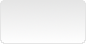# Latex, PgfPlots - Filling areas under and between curves## Introduction

In some circumstances, areas need to be highlighted. How to achieve it using Latex and PgfPlots package ?

$$A = \int_{-2}^0 \left[f(x) -g(x)\right] \ dx + \int_{0}^2 \left[g(x) -f(x)\right] \ dx = 24$$In the above graph, we want to fill the areas between the two curves. The Tex code for the 2 graphs in a tikzpicture environment is :

\documentclass[tikz]{standalone}

\usepackage{pgfplots}
\usepackage{amsmath,amssymb,amsfonts}
\usepgfplotslibrary{fillbetween}

\begin{document}

\begin{tikzpicture}

\begin{axis}[
xmin=-3, xmax=3,
ymin=-10, ymax=10,
xtick distance=1, ytick distance=4 ]

{3*x^3 - x^2 - 10*x};

{- x^2 + 2*x};

\draw [dashed, opacity=0.4] (axis cs:{-2,0}) -- (axis cs:{-2,-8});

\node[color=red, font=\footnotesize] at (axis cs: -1.6,7) {$f(x)=3x^3 - x^2 - 10x$};
\node[color=blue, font=\footnotesize] at (axis cs: 1.1,2.2) {$g(x)=- x^2 + 2x$};

\end{axis}

\end{tikzpicture}

\end{document}

## Library fillbetween, areas between 2 curves

• To fill the area between the 2 curves : import the fillbetween PgfPlots library
\usepgfplotslibrary{fillbetween}
• Give a name path to each plot, using name path option : name paths curves are named f and g in this example.
\addplot [domain=-2.5:2.5, samples=100, name path=f, thick, color=red!50]
{3*x^3 - x^2 - 10*x};

\addplot [domain=-2.5:2.5, samples=100, name path=g, thick, color=blue!50]
{- x^2 + 2*x}; 
• Add a plot to fill the area using fill between [of=<graph name path 1> and <graph name path 2>]
\addplot[red!10, opacity=0.4] fill between[of=f and g, soft clip={domain=-2:2}];
The soft clip option is used to restrict the domain.

That’s all.\documentclass[tikz]{standalone}

\usepackage{pgfplots}
\usepackage{amsmath,amssymb,amsfonts}
\usepgfplotslibrary{fillbetween}

\begin{tikzpicture}

\begin{axis}[
xmin=-3, xmax=3,
ymin=-10, ymax=10,
xtick distance=1, ytick distance=4 ]

\addplot [domain=-2.5:2.5, samples=100, name path=f, thick, color=red!50]
{3*x^3 - x^2 - 10*x};

\addplot [domain=-2.5:2.5, samples=100, name path=g, thick, color=blue!50]
{- x^2 + 2*x};

\addplot[red!10, opacity=0.4] fill between[of=f and g, soft clip={domain=-2:2}];

\draw [dashed, opacity=0.4] (axis cs:{-2,0}) -- (axis cs:{-2,-8});

\node[color=red, font=\footnotesize] at (axis cs: -1.6,7) {$f(x)=3x^3 - x^2 - 10x$};
\node[color=blue, font=\footnotesize] at (axis cs: 1.1,2.2) {$g(x)=- x^2 + 2x$};

\end{axis}

\end{tikzpicture}

\end{document}

## Areas and x axis

To fill areas between a curve and the x axis, create a named path for the horizontal axis using path command :

\addplot [domain=-2.5:2.5, samples=100, name path=f, thick, color=red!50]
{3*x^3 - x^2 - 10*x};

\path [name path=xaxis]
(\pgfkeysvalueof{/pgfplots/xmin},0) --
(\pgfkeysvalueof{/pgfplots/xmax},0);

\addplot[red!10, opacity=0.4] fill between [of=f and xaxis, soft clip={domain=-2:2}];## Fillbetween, split. Area segments

Using fill between, the area between 2 curves can be splitted into segments depending on every intersection of the two curves. An index is applied to each area segment. The split option is mandatory to compute segments.

\addplot [domain=-2.5:2.5, samples=100, name path=f, thick, color=red!50]
{3*x^3 - x^2 - 10*x};

\path [name path=xaxis]
(\pgfkeysvalueof{/pgfplots/xmin},0) --
(\pgfkeysvalueof{/pgfplots/xmax},0);

every odd segment/.style ={green!20!white}];every segment no 2/.style={pattern=north east lines}
every last segment/.style={top color=orange, bottom color=blue}